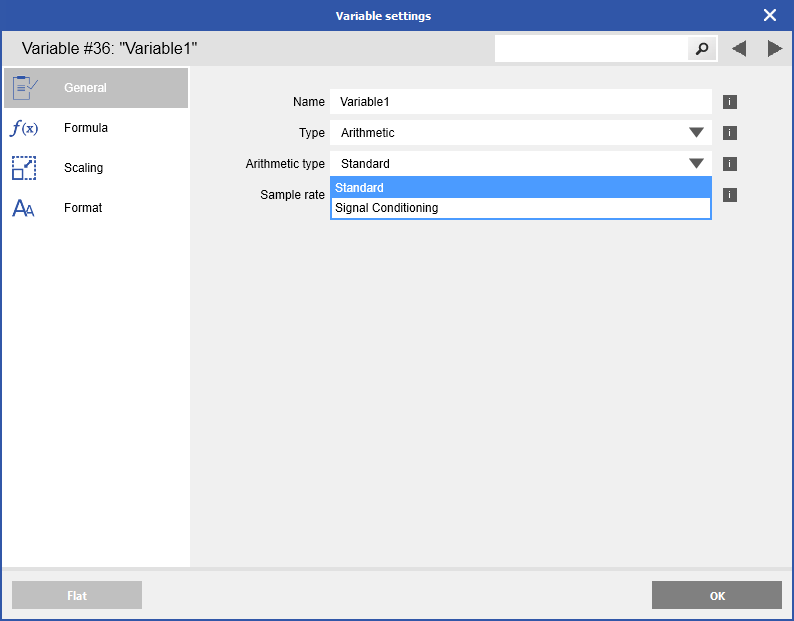# Arithmetic functions: standard vs. signal conditioning

## The Q.series X I/O modules separate between standard arithmetic functions and signal conditioning arithmetic functions. Why is this?

### For Q.series X I/O modules the same functions (e.g. selRMS, max, or min) can be configured as standard or as signal conditioning arithmetic variables.The difference is that the standard arithmetic variables are calculated in the DSP and the signal conditioning arithmetic variables in the FPGA. The standard arithmetic variables calculate their result out of the copy read by the DSP from the FPGA actual value.

### Standard arithmetic functions

• +
• -
• *
• /
• min()
• max()
• selRMS(Vx; Ts) ... Ts = integration time (period of the processed signal)
• integ()
• spec1()
• spec2()
• spec3()
• spec4()

### Signal conditioning arithmetic functions

These functions can be used to get intermediate results from the analog input or remote variables calculated inside the FPGA.

• min() … min value of an analog or remote input
• max() … max value of an analog or remote input